# Join Multiple data.tables in R (6 Examples)

This article explains how to merge multiple data.tables in various ways in R programming. We show the different possible ways of merging data.tables with two and three data.tables.

So now the part you have been waiting for – the examples.

## Example Data & Packages

We first have to install and load the data.table package:

```install.packages("data.table")                    # Install & load data.table package
library("data.table")```

We also have to create some data that we can use in the example syntax later on:

```DT1 <- data.table(V1 = 1:4,                       # Create data.table
V2 = c("a", "a", "b", "b"),
V3 = c("c", "d", "c", "d"))
DT1                                               # Print data.table```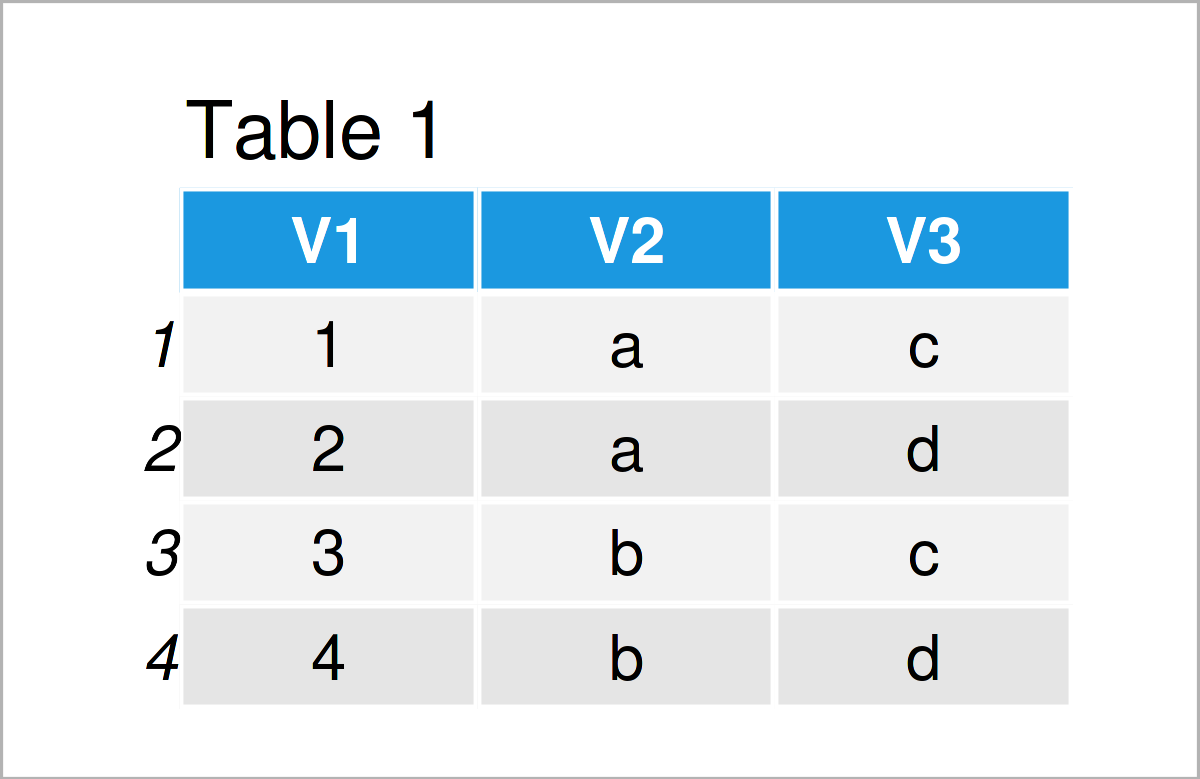Table 1 shows the structure of the exemplifying data: It is made of four rows and three columns.

```DT2 <- data.table(V1 = 2:5,                       # Create data.table
V4 = 5:6,
V5 = c(FALSE, TRUE, TRUE, TRUE))
DT2                                               # Print data.table```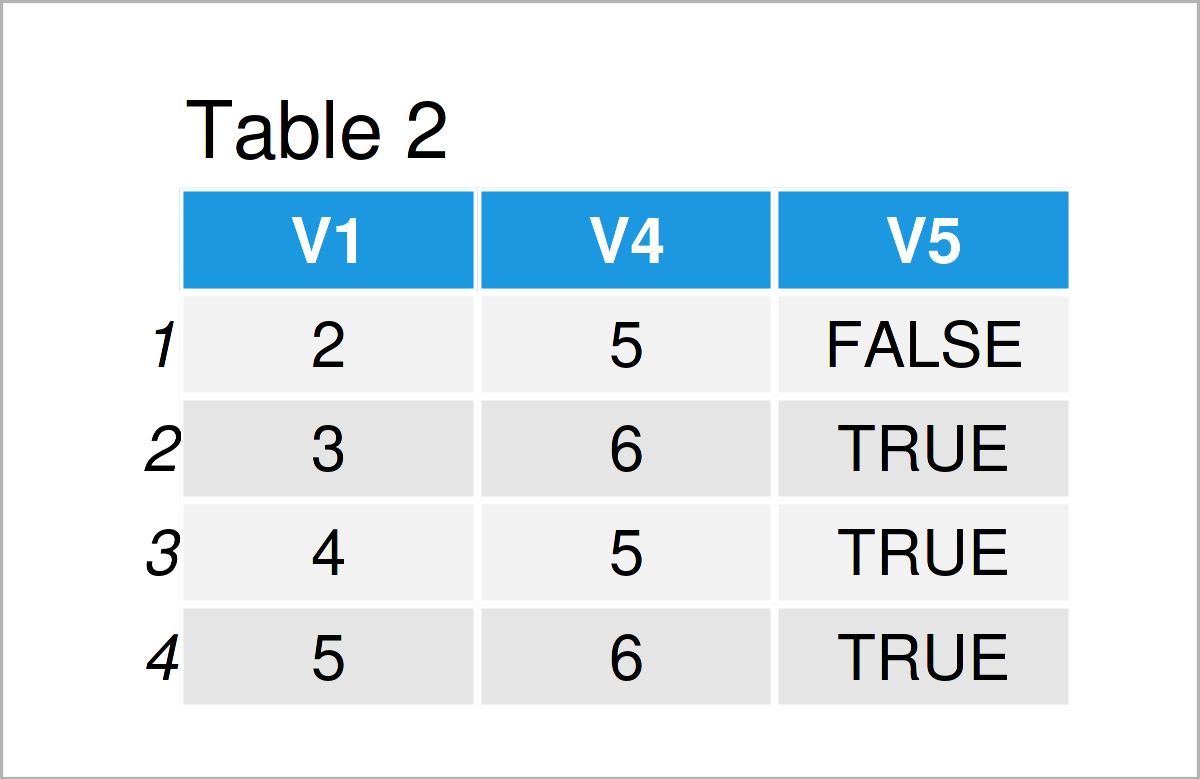In Table 2 it is shown that we have created a data.table with the previous R programming syntax. Take a look at variable V1, we can see this variable as an identifier. Comparing DT1 to DT2 we see that DT1 and DT2 share information on observations with V1=2,3,4.

Finally, we generate a third data.table called DT3.

```DT3 <- data.table(V1 = 4:6, # Create data.table
V2 = c("b", "b", "a"),
V6 = c(10, 11, 12))
DT3 # Print data.table```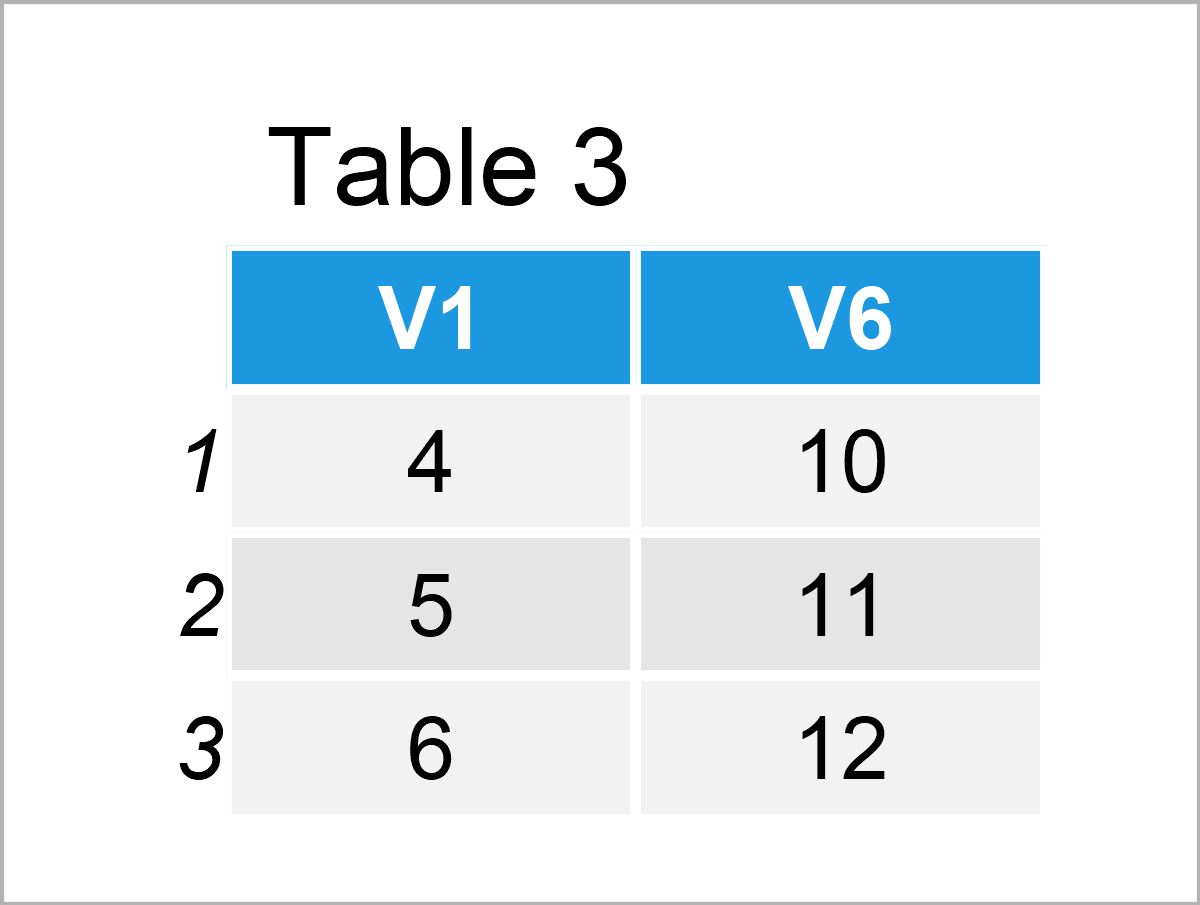As you can see from Table 3, we again have a column V1 to identify observations in the three data.tables DT1, DT2, and DT3.

To get further information on data.table: Take a look at our data.table page here for more data.table tutorials. If you want to have a more visual intuition of the different ways of combining data.tables in R, have a look at this R Studio post. Furthermore, you can find the CRAN page of the package here and the documentation here.

## Example 1: Inner Join of Two data.tables

In Example 1, I’ll demonstrate how to make an inner join of two data.tables with merge().

```DT1_2_inner <- merge(DT1, DT2, all = FALSE)       # Inner join
DT1_2_inner                                       # Print data.table```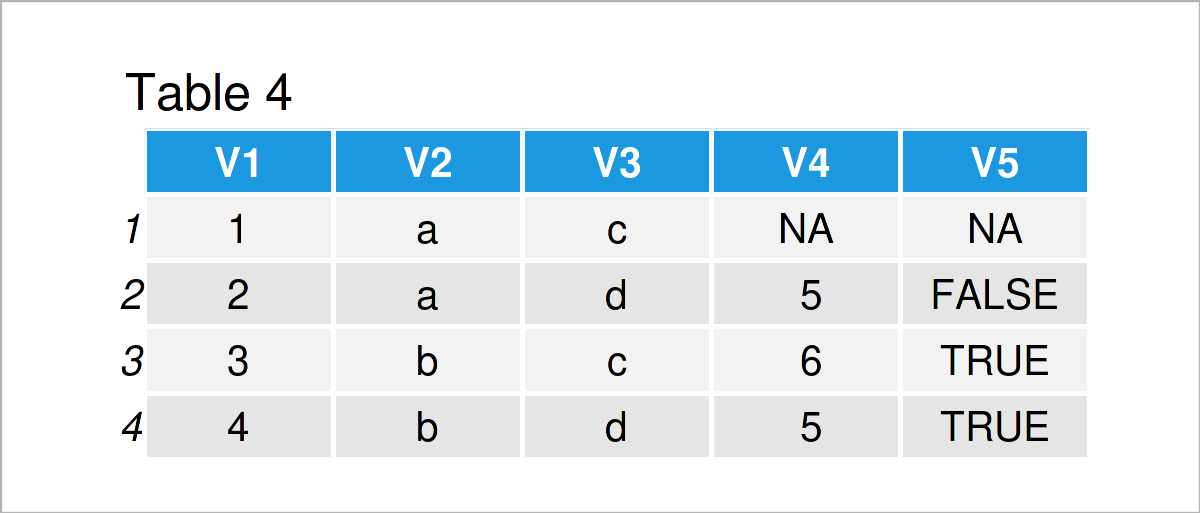Table 4 shows the output of the previous syntax – the inner join. Function merge() automatically takes the common variable V1 as the identifier variable of the two data.tables. As we set argument all = FALSE, we indicate that we only want to merge those data rows with the common values of identifier V1.

## Example 2: Left Outer Join of Two data.tables

In this section, I’ll illustrate how to make a left outer join.

```DT1_2_out_left <- merge(DT1, DT2, all.x = TRUE)   # Outer join: Left
DT1_2_out_left                                    # Print data.table```After executing the previously shown syntax the data.table shown in Table 5 has been created. We made a left outer join. That is, we take DT1 and then add all additional information of these data rows (identified with common variable V1) available from DT2.

## Example 3: Right Outer Join of Two data.tables

The following R programming syntax explains how to do a right join.

```DT1_2_out_right <- merge(DT1, DT2, all.y = TRUE)  # Outer join: Right
DT1_2_out_right                                   # Print data.table```The output of the previous R code is shown in Table 6. This time we took the information of DT2 and merged to it the information of DT1. All unavailable information is set to NA.

## Example 4: Full Join of Two data.tables

In this example, I’ll explain how to do a full join of two data.tables.

```DT1_2_full <- merge(DT1, DT2, all = TRUE)         # Full join
DT1_2_full                                        # Print data.table```Table 7 shows the output of the previous R programming syntax. With argument all = TRUE we indicate that we want to merge all available information from the two datasets. All missing information is set to NA.

## Example 5: Full Join of Three data.tables

The following code shows how to merge more than two data.tables at the same time. In the example, we conduct a full join, merging all information from three data.tables. Remember: It is important that we have a common variable V1, indicating how to match the information.

```DT1_2_3_full <- Reduce(function (...) { merge(..., all = TRUE) },   # Full join
list(DT1, DT2, DT3))
DT1_2_3_full                                                        # Print data.table```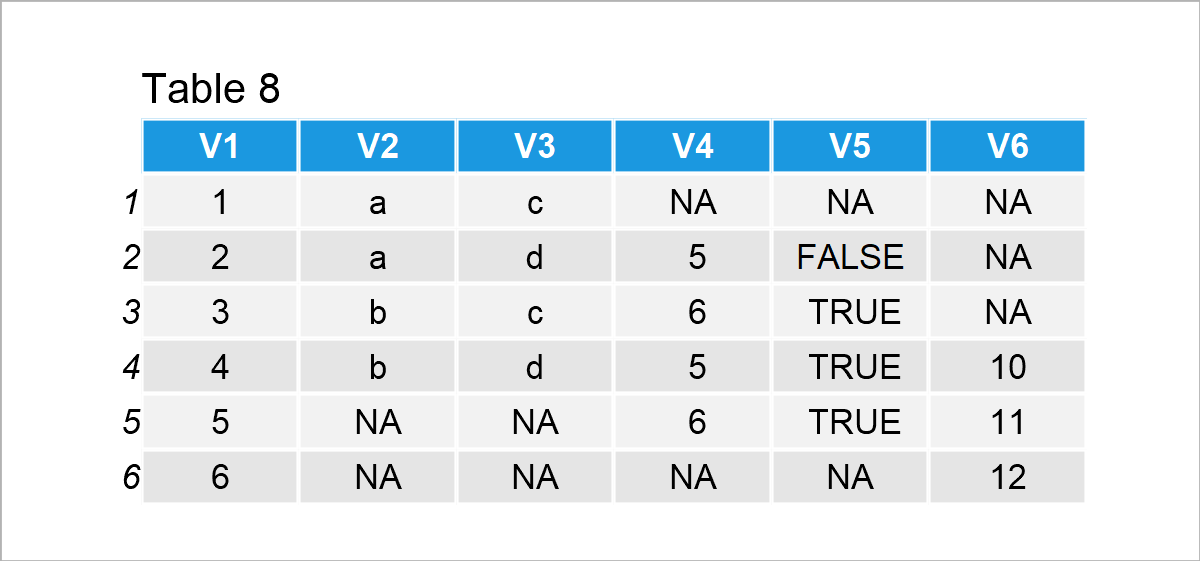The resulting merged data.table is shown in Table 8.

## Example 6: Inner Join of Three data.tables

As a last example, we make an inner join of three data.tables. For that, we simply set argument all = FALSE in the merge() function.

```DT1_2_3_inner <- Reduce(function (...) { merge(..., all = FALSE) },   # Inner join
list(DT1, DT2, DT3))
DT1_2_3_inner                                                         # Print data.table```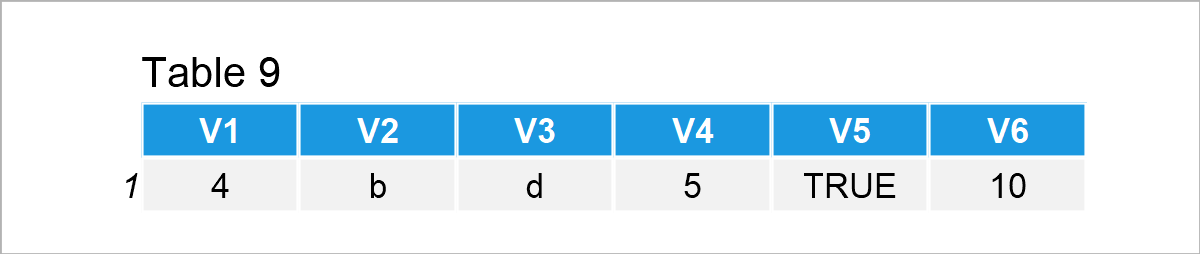You can see that the three data.tables only have V1=4 in common.

## Video, Further Resources & Summary

Do you want to learn more about the merging of data.tables in R? Then I recommend having a look at the following video on my YouTube channel. In the video, I demonstrate the R programming codes of this tutorial:

Furthermore, you could have a look at the related tutorials on this homepage:

You have learned in this article how to combine data.tables in R. If you have any additional comments and/or questions, don’t hesitate to let me know in the comments section.

This page was created in collaboration with Anna-Lena Wölwer. Have a look at Anna-Lena’s author page to get further information about her academic background and the other articles she has written for Statistics Globe.

Subscribe to the Statistics Globe Newsletter